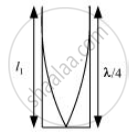CBSE (Science) Class 11CBSE
Share

# A Metre-long Tube Open at One End, with a Movable Piston at the Other End, Shows Resonance with a Fixed Frequency Source (A Tuning Fork of Frequency 340 Hz) When the Tube Length is 25.5 Cm Or 79.3 Cm. Estimate the Speed of Sound in Air at the Temperature of the Experiment. the Edge Effects May Be Neglected. - CBSE (Science) Class 11 - Physics

ConceptThe Speed of a Travelling Wave

#### Question

A metre-long tube open at one end, with a movable piston at the other end, shows resonance with a fixed frequency source (a tuning fork of frequency 340 Hz) when the tube length is 25.5 cm or 79.3 cm. Estimate the speed of sound in air at the temperature of the experiment. The edge effects may be neglected.

#### Solution 1

Frequency of the turning fork, ν = 340 Hz

Since the given pipe is attached with a piston at one end, it will behave as a pipe with one end closed and the other end open, as shown in the given figure.Such a system produces odd harmonics. The fundamental note in a closed pipe is given by the relation:

l_1 = lambda/4

Where,

Length of the pipe, l_1 = 25.5 cm = 0.255 m

:.lambda = 4l_1 = 4xx 0.255 = 1.02 m

The speed of sound is given by the relation: v = vlambda = 340 × 1.02 = 346.8 m/s

#### Solution 2

Frequency of nth mode of vibration of the closed organ pipe of lenght

l_1 = (2n - 1) v/(4l_1)

Frequency of (n+1) th mode of vibration of closed pipe of length

'l_2' = [2(n+1)-1] v/(4l_2) = (2n + 1) v/(4l_2)

Both the modes are given to resonate with a frequency of 340 Hz.

:. (2n - 1) v/(4l_1) = (2n+1) v/(4l_2)

or (2n -1)/(2n+2) = l_1/l_2 = 25.5/79.3 =  1/3

[Approximation has been used because edge effect is being ignored. Moreover, we know that in the case of a closed organ pipe, the second resonance length is three times the first resonance length.]
On simplification, n = 1

Now, (2n-1)υ/4l1 = 340. Substituting values

(2 x 1 -1) υ x 100/4 x 25.5 = 340 or υ =346.8 ms-1

Is there an error in this question or solution?

#### APPEARS IN

Solution A Metre-long Tube Open at One End, with a Movable Piston at the Other End, Shows Resonance with a Fixed Frequency Source (A Tuning Fork of Frequency 340 Hz) When the Tube Length is 25.5 Cm Or 79.3 Cm. Estimate the Speed of Sound in Air at the Temperature of the Experiment. the Edge Effects May Be Neglected. Concept: The Speed of a Travelling Wave.
S